NEET  >  Test: Free Body - 1

# Test: Free Body - 1

Test Description

## 10 Questions MCQ Test Topic-wise MCQ Tests for NEET | Test: Free Body - 1

Test: Free Body - 1 for NEET 2022 is part of Topic-wise MCQ Tests for NEET preparation. The Test: Free Body - 1 questions and answers have been prepared according to the NEET exam syllabus.The Test: Free Body - 1 MCQs are made for NEET 2022 Exam. Find important definitions, questions, notes, meanings, examples, exercises, MCQs and online tests for Test: Free Body - 1 below.
Solutions of Test: Free Body - 1 questions in English are available as part of our Topic-wise MCQ Tests for NEET for NEET & Test: Free Body - 1 solutions in Hindi for Topic-wise MCQ Tests for NEET course. Download more important topics, notes, lectures and mock test series for NEET Exam by signing up for free. Attempt Test: Free Body - 1 | 10 questions in 10 minutes | Mock test for NEET preparation | Free important questions MCQ to study Topic-wise MCQ Tests for NEET for NEET Exam | Download free PDF with solutions
 1 Crore+ students have signed up on EduRev. Have you?
Test: Free Body - 1 - Question 1

### Force that produces an acceleration of 1 ms−2 in a body of mass of 1 kg is called

Detailed Solution for Test: Free Body - 1 - Question 1

We know that F = ma, and for m = 1kg and a = 1m/s2
We get F = 1N which is one newton

Test: Free Body - 1 - Question 2

### A horizontal force of 100 N pulls two masses 5 kg and 10 kg tied to each other by a light string. What is the tension in the string if the force is applied on 10 kg mass?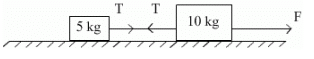Detailed Solution for Test: Free Body - 1 - Question 2

At first considering both blocks as one system with only one external force F
We get common acceleration at right be a = 100/15 m/s2
Now considering 10 kg block
We get F - T = 10a
i.e. T = 100  - 10(100/15)
= 100 (1 - 2/3)
= 33.33 N

Test: Free Body - 1 - Question 3

### A man weighing 100 kgf carries a load of 10 kgf on his head. He jumps from tower with that load. What will be the weight of load experienced by the man.

Test: Free Body - 1 - Question 4

For a body to be able to loop a vertical circle of radius R, the minimum velocity required at its lowest point is:

Detailed Solution for Test: Free Body - 1 - Question 4

For a body able to loop a vertical circle, the tension in the string must not be lesser than zero at the highest point. Hence when the tension at the highest point is zero we will obtain the minimum velocity. I.e.
At the highest point as T = 0, hence we get
Mg = Mv2/R
(comparing the centripetal acceleration with weight, where R is radius of vertical circle)
Hence we get the minimum velocity at the topmost point to be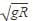Thus if we apply the work energy theorem upon the particle from its lowermost point to its top most point, we get net displacement as 2R and thus minimum velocity at lowermost point to be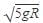Test: Free Body - 1 - Question 5

A block of 5 kg mass rests on a horizontal floor. The action of the block on the floor is

Detailed Solution for Test: Free Body - 1 - Question 5

Weight of the block, mg = 5kg x 10 m/s2 = 50 N.
According to Newton’s third law, the action of the block, that is the force exerted on the floor by the block is equal to 50 N in magnitude and is directly vertically downward.

Test: Free Body - 1 - Question 6

A lift is moving down with the acceleration 3 m/s2. A ball is released 1.7 m above the the lift floor. How long will it take to hit the lift floor.

Detailed Solution for Test: Free Body - 1 - Question 6

As the lift itself is accelerating hence the net downwards acceleration of the ball will be 9.8 - 3
= 6.8 m/s2
Thus by applying second equation of motion we get, t =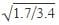= 0.71

Test: Free Body - 1 - Question 7

A body of mass 2 kg is hung on a spring balance mounted vertically in a lift. If the lift moves up with an acceleration equal to the acceleration due to gravity, the reading on the spring balance will be

Detailed Solution for Test: Free Body - 1 - Question 7

As the lift moves upwards but the spring feels itself at rest hence we need to compensate the non inertial frame by adding an appropriate pseudo force to treat it as an inertial frame. Hence the pseudo force to be applied acts on every mass in the lift which is equal to mass x acceleration (=g) downwards.
Hence the tension in the spring would be 40N (20 due to weight and 20 pseudo). Thus the reading would be 4kg.

Test: Free Body - 1 - Question 8

A block of mass m is pulled by applying a force F at an angle θ with the horizontal surface. Its acceleration will be given by

Detailed Solution for Test: Free Body - 1 - Question 8

When the force F is applied at some angle, only the component of it which is parallel to the surface would provide acceleration to it. The other one would be balanced by the normal force. Hence as the component parallel to the surface is F.cosΘ , we get a = F.cosΘ / m

Test: Free Body - 1 - Question 9

Consider two blocks of masses m1 and m2 placed in contact with each other on a friction less horizontal surface. Let a force F be applied on block of mass m1, then the value of contact force between the blocks is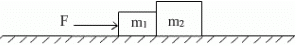Detailed Solution for Test: Free Body - 1 - Question 9

At first consider both blocks as one system then we get the common acceleration of the system,  a =  F / m1 + m2
Now if we see only mass m1
We get that for contact force f,
F - f = m1a
Thus we get f = F - m1 F / m1 + m2
=  m2F /  m1 + m2

Test: Free Body - 1 - Question 10

The couple and the other two force systems in free body diagrams can be easily simplified.

Detailed Solution for Test: Free Body - 1 - Question 10

Both of them are vector quantities. And both of them can be easily simplified. If taken in the vector form then the task is even easier. Thus it is not necessary for the force or the couple to be vector only, even if the magnitude is taken, the simplification is done in the 2D.

## Topic-wise MCQ Tests for NEET

1493 tests
 Use Code STAYHOME200 and get INR 200 additional OFF Use Coupon Code
Information about Test: Free Body - 1 Page
In this test you can find the Exam questions for Test: Free Body - 1 solved & explained in the simplest way possible. Besides giving Questions and answers for Test: Free Body - 1, EduRev gives you an ample number of Online tests for practice

## Topic-wise MCQ Tests for NEET

1493 tests

### How to Prepare for NEET

Read our guide to prepare for NEET which is created by Toppers & the best Teachers